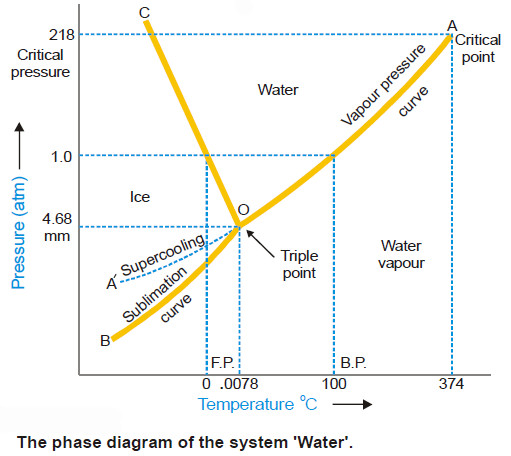# Water system, Phase diagram of Water

## The Water system

– Under normal conditions the Water system is a three-phase, one-component system.

– The three phases involved are liquid water, ice, water vapour.

– All these phases can be represented by one chemical entity H2O and hence one component of the system.

– The number of phases which can exist in equilibrium any time depends on the conditions of temperature and pressure.

– The phase diagram or PT-graph of the system/ water/ice/vapour is shown in the following Figure.– The salient features of the phase diagram are listed below.

(1) The Curves OA, OB, OC

(2) The Triple Point O

(3) The Areas AOC, AOB, BOC

– Let us proceed to discuss the significance of each of these features.

### (1) The Curves OA, OB, OC

– These three curves meet at the point O and divide the diagram into three regions or areas.

Curve OA, the Vapour Pressure curve of Water.

– It represents the vapour pressure of liquid water at different temperatures.

– The two phases water and water vapour coexist in equilibrium along this curve.

– The curve OA terminates at A, the critical point (218 atm, temp. 374ºC) when the liquid and vapour are indistinguishable from each other and there is left one phase only.

– When the vapour pressure is equal to one-atmosphere, the corresponding temperature, as indicated on the phase diagram is the boiling point (100ºC) of water.

Curve OB, the Sublimation curve of Ice

– It shows the vapour pressure of solid ice at different temperatures.

– The two phases solid ice and vapour coexist in equilibrium along this curve.

– At the lower limit the curve OB terminates at absolute zero (– 273ºC) where no vapour exists.

Curve OC, the Fusion curve of Ice

– It depicts the effect of pressure on the melting point of ice.

– Here ice and water coexist in equilibrium.

– The fact that OC slopes to the left indicates that the melting point of ice decreases with increase of pressure.

– Since ice melts with decrease in volume by Le Chatelier’s principle the melting point is lowered by an increase of pressure.

– It may be noted that the 1.0 atmosphere line meets the fusion curve at 0ºC which is the normal melting point of ice.

– Along the curves OA, OB, OC there are two phases in equilibrium and one component. Therefore,

F = C – P + 2 = 1 – 2 + 2 = 1

– Hence each two-phase system :

• water/water vapour represented by OA
• ice/water vapour represented by OB
• ice/water represented by OC

has one degree of freedom i.e., is monovariant.

### (2) The Triple point (O)

The curves OA, OB and OC meet at the triple point ‘O’ where all the three phases liquid water/ ice/vapour are in equilibrium.

– This occurs at 0.0076ºC and vapour pressure 4.58 mm Hg. Since there are three phases and one component, we have

F = C– P + 2 = 1 – 3 + 2 = 0

i.e., the system at the triple point is nonvariant.

– Thus if either pressure or temperature is changed, the three phases would not exist and one of the phases would disappear.

### (3) Area AOC, AOB, BOC

The areas or regions between the curves show the conditions of temperature and pressure under which a single phase–ice, water or vapour is capable of stable existence. Thus,

– Area AOC represents conditions for the one-phase system water.

– Area AOB represents conditions for the one-phase system water vapour.

– but Area BOC represents conditions for the one-phase system ice.

In all the three areas there being one-phase and one-component, we have:

F = C – P + 2 = 1 – 1 + 2 = 2

– Thus each system water, water vapour, or ice has 2 degrees of freedom i.e., the system is
bivariant.

### (4) Metastable system : Supercooled Water/Vapour System

– The vapour pressure curve of water AO can be continued past the triple point as shown by the dashed line OA’.

– That is, water can be supercooled by carefully eliminating solid particles.

– The supercooled water/vapour system is metastable (unstable).

– It at once reverts to the stable system ice/vapour on the slightest disturbance or introducing a crystal of ice.